# ski-in / ski-out - Page 31

Viewing mode
743 results
• Slopes access :
• 50 -300m to the slopes
• Rooms :
• 3 rooms
• Total surface (sq.m) :
• 50 sq.m
•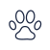• Garage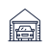•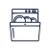•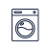•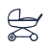• Slopes access :
• 50 -300m to the slopes
• Rooms :
• Duplex
• Total surface (sq.m) :
• 55 sq.m
••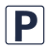••• Slopes access :
• ski-in (-50 m)
• Slopes access :
• ski-in (-50 m)
• Rooms :
• Duplex
• Total surface (sq.m) :
• 60 sq.m
•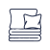•••• Slopes access :
• ski-in (-50 m)
• Rooms :
• Studio
• Studio + cabin(s)
• Total surface (sq.m) :
• 32 sq.m
••••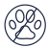• Slopes access :
• ski-in (-50 m)
• Slopes access :
• ski-in (-50 m)
• Rooms :
• 2 rooms
• Slopes access :
• ski-in (-50 m)
• Rooms :
• 3 rooms
• Total surface (sq.m) :
• 42 sq.m
••••• Slopes access :
• ski-in (-50 m)
• Rooms :
• 3 rooms
• Total surface (sq.m) :
• 42 sq.m
•••••• Slopes access :
• ski-in (-50 m)
• Rooms :
• 3 rooms
• Total surface (sq.m) :
• 55 sq.m
•••••• Slopes access :
• 50 -300m to the slopes
• Rooms :
• 5 rooms
• Total surface (sq.m) :
• 97 sq.m
•••••• Slopes access :
• ski-in (-50 m)
• Rooms :
• Studio
• Studio + cabin(s)
• Total surface (sq.m) :
• 31 sq.m
••••• Slopes access :
• ski-in (-50 m)
• Rooms :
• 2 rooms
• 2 rooms + cabin(s)
• Total surface (sq.m) :
• 40 sq.m
••••• Slopes access :
• ski-in (-50 m)
• Rooms :
• Studio
• Total surface (sq.m) :
• 30 sq.m
•••• Slopes access :
• 50 -300m to the slopes
• Rooms :
• 4 rooms
• 4 rooms + cabin(s)
• Total surface (sq.m) :
• 103 sq.m
•••• Slopes access :
• ski-in (-50 m)
• Rooms :
• Studio
• Studio + cabin(s)
• Total surface (sq.m) :
• 25 sq.m
••••• Slopes access :
• 50 -300m to the slopes
• Rooms :
• 3 rooms
• Total surface (sq.m) :
• 44 sq.m
••••• Slopes access :
• ski-in (-50 m)
• Rooms :
• Studio + cabin(s)
• Total surface (sq.m) :
• 29 sq.m
••••• Slopes access :
• 50 -300m to the slopes
• Rooms :
• 2 rooms
• 2 rooms + cabin(s)
• Total surface (sq.m) :
• 36 sq.m
•••• Slopes access :
• ski-in (-50 m)
• Rooms :
• Studio
• Total surface (sq.m) :
• 25 sq.m
••••